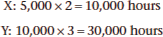# Cost-Plus Pricing

A pricing method in which selling price of a product is calculated by adding a profit margin to the cost of the product. This method is known as Cost-Plus pricing method.

The general approach to pricing is that all costs should be recovered and therefore included when calculating the price. However, to create a profit margin a mark-up will be added to the cost of the product or service. Recovering costs, both direct and indirect, and adding a mark-up is a long-term pricing strategy. It is worth noting that cost-plus pricing is also referred to as full-cost pricing.

Formula

The product price using Cost plus Pricing is determined as follows:

Price = Cost × (1 + Profit Margin Percentage)

Whereas the profit is a fixed amount per unit of the product:

Price = Profit + Cost

## Cost-Plus Pricing Example

Wearham Ltd produces and sells two products. The products are priced on a cost-plus pricing basis. The following table shows the production costs of the two products:

 Product X Product Y Demand 5,000 units 10,000 units \$/unit \$/unit Direct materials 15 20 Direct labor (\$10 per hour) 20 30 Variable production costs 10 15 Fixed production costs per unit 6 9 Total production costs 51 74

The company’s policy is to allocate 10 per cent of the total production costs for selling and administration overheads and a further 15 per cent mark-up for profit based on total cost.

Variable overheads are directly related to direct labor costs and fixed overheads are absorbed on the basis of labor hours.

Wearham Ltd are considering producing 10,000 units of product Z which will have material costs of \$10 and will use 2 labor hours to produce per unit.

Required:

Calculate the selling price of product Z on a full cost basis. (You should work to two decimal places.) This question requires you to examine the current product portfolio, X and Y and analyzes the changes product Z would have on the cost structure of the three products.

 Workings/ Notes Product Z Demand 10,000 units \$/unit Direct material A 10 Direct labor (\$10 per hour) B 20 Variable production costs C 10 Fixed production costs D 4 Total production costs E 44.00 Selling & Admin. Overheads F 4.40 Total cost G 48.40 Profit Margin H 7.26 Selling price I 55.66

Solution:

a) The material costs are provided in the question.

b) In the cost structure of X and Y the question states that the cost per direct labor hour is 10 dollars per hour, the questions states that product Z will require two hours in production. Therefore 10 × 2 hrs. = \$20.00.

c) The question states there is a direct relationship between variable cost and labor hours therefore the variable overhead cost is calculated as follows: Relationship is 20/10 = 2 (using data for X), test 30/15 = 2 (using data for Y).

The variable overhead cost for Z is 20/2 = 10.

d) Fixed overhead costs need more consideration. The question does not indicate that any additional fixed costs will be introduced due to the new product therefore the current fixed costs need to be allocated across the three products: X, Y and Z.

We need to first examine how many labor hours are used in total for X and Y because the question states that fixed costs are absorbed on the basis of labor hours. Total labor hours when just X and Y are being produced:Which means the total labor hours = 40,000 hours (10,000 + 30,000).

The overhead rate at the moment is 6/2 = \$3. This can be established by examining one of the current products such as X. Product X uses two labor hours for the production of one unit, you can calculate this by taking the labor cost and dividing it by the labor cost per hour \$20/\$10 per hour.

The total fixed overhead cost would be \$3 × 40,000 hours = \$120,000.

As fixed costs remain constant when producing Z the new overhead rate would be:

Labor hours for X and Y: (40,000 plus labor hours for Z: 2 × 10,000) = 60,000 hours.

New rate: \$120,000/60,000 hours = \$2 per labor hour.

Therefore fixed overhead rate for Z would be \$2 × 2 hrs. = \$4.

e) Total production cost is simply the direct material, direct labor, variable cost and fixed cost.

f) In the question it states that the company has a policy to add 10 per cent of the total production cost to cover selling and administrations costs. Z would be \$44.00 × 0.1 = \$4.40.

g) Total cost is the production cost plus the selling and administration cost.

h) The question tells you that the company policy is to add 15 per cent on total cost as a mark-up. Z would require the total cost of \$48.40 being multiplied by 15 per cent which is \$48.40 × 0.15 = \$7.26.

i) The selling price is the total cost plus the mark-up which is \$55.66.